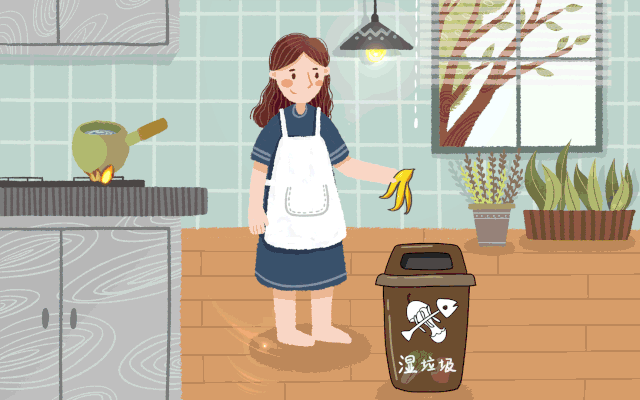﻿ 垃圾分类·青春先行丨分类时代已到，这些知识你要懂！ - 福州新闻网

# 垃圾分类·青春先行丨分类时代已到，这些知识你要懂！

“你是什么垃圾？”' data-lazy='1' data-height='44' width='49' height='auto'>' data-lazy='1' data-height='400' data-width='640' width='640' height='auto'>

▲湿垃圾桶' data-lazy='1' data-height='400' data-width='640' width='640' height='auto'>

▲干垃圾桶' data-lazy='1' data-height='13' width='483' height='auto'>' data-lazy='1' data-height='39' width='61' height='auto'>垃圾分为四类' data-lazy='1' data-height='550' data-width='640' width='640' height='auto'>' data-lazy='1' data-height='734' data-width='504' width='504' height='auto'>' data-lazy='1' data-height='759' data-width='516' width='516' height='auto'>' data-lazy='1' data-height='734' data-width='487' width='487' height='auto'>' data-lazy='1' data-height='255' data-width='477' width='477' height='auto'>' data-lazy='1' data-height='13' width='483' height='auto'>' data-lazy='1' data-height='317' data-width='500' width='500' height='auto'>